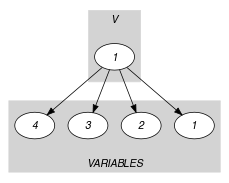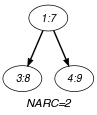## 5.276. next_greater_element

Origin

M. Carlsson

Constraint

$\mathrm{𝚗𝚎𝚡𝚝}_\mathrm{𝚐𝚛𝚎𝚊𝚝𝚎𝚛}_\mathrm{𝚎𝚕𝚎𝚖𝚎𝚗𝚝}\left(\mathrm{𝚅𝙰𝚁}\mathtt{1},\mathrm{𝚅𝙰𝚁}\mathtt{2},\mathrm{𝚅𝙰𝚁𝙸𝙰𝙱𝙻𝙴𝚂}\right)$

Arguments
 $\mathrm{𝚅𝙰𝚁}\mathtt{1}$ $\mathrm{𝚍𝚟𝚊𝚛}$ $\mathrm{𝚅𝙰𝚁}\mathtt{2}$ $\mathrm{𝚍𝚟𝚊𝚛}$ $\mathrm{𝚅𝙰𝚁𝙸𝙰𝙱𝙻𝙴𝚂}$ $\mathrm{𝚌𝚘𝚕𝚕𝚎𝚌𝚝𝚒𝚘𝚗}\left(\mathrm{𝚟𝚊𝚛}-\mathrm{𝚍𝚟𝚊𝚛}\right)$
Restrictions
 $\mathrm{𝚅𝙰𝚁}\mathtt{1}<\mathrm{𝚅𝙰𝚁}\mathtt{2}$ $|\mathrm{𝚅𝙰𝚁𝙸𝙰𝙱𝙻𝙴𝚂}|>0$ $\mathrm{𝚛𝚎𝚚𝚞𝚒𝚛𝚎𝚍}$$\left(\mathrm{𝚅𝙰𝚁𝙸𝙰𝙱𝙻𝙴𝚂},\mathrm{𝚟𝚊𝚛}\right)$
Purpose

$\mathrm{𝚅𝙰𝚁}\mathtt{2}$ is the value strictly greater than $\mathrm{𝚅𝙰𝚁}\mathtt{1}$ located at the smallest possible entry of the table $\mathrm{𝚃𝙰𝙱𝙻𝙴}$. In addition, the variables of the collection $\mathrm{𝚅𝙰𝚁𝙸𝙰𝙱𝙻𝙴𝚂}$ are sorted in strictly increasing order.

Example
$\left(7,8,〈3,5,8,9〉\right)$

The $\mathrm{𝚗𝚎𝚡𝚝}_\mathrm{𝚐𝚛𝚎𝚊𝚝𝚎𝚛}_\mathrm{𝚎𝚕𝚎𝚖𝚎𝚗𝚝}$ constraint holds since:

• $\mathrm{𝚅𝙰𝚁}\mathtt{2}$ is fixed to the first value 8 strictly greater than $\mathrm{𝚅𝙰𝚁}\mathtt{1}=7$,

• The $\mathrm{𝚟𝚊𝚛}$ attributes of the items of the collection $\mathrm{𝚅𝙰𝚁𝙸𝙰𝙱𝙻𝙴𝚂}$ are sorted in strictly increasing order.

Typical
 $|\mathrm{𝚅𝙰𝚁𝙸𝙰𝙱𝙻𝙴𝚂}|>1$ $\mathrm{𝚛𝚊𝚗𝚐𝚎}$$\left(\mathrm{𝚅𝙰𝚁𝙸𝙰𝙱𝙻𝙴𝚂}.\mathrm{𝚟𝚊𝚛}\right)>1$
Usage

Originally introduced in [CarlssonBeldiceanu04] for modelling the fact that a nucleotide has to be consumed as soon as possible at cycle $\mathrm{𝚅𝙰𝚁}\mathtt{2}$ after a given cycle $\mathrm{𝚅𝙰𝚁}\mathtt{1}$.

Remark

Similar to the $\mathrm{𝚖𝚒𝚗𝚒𝚖𝚞𝚖}_\mathrm{𝚐𝚛𝚎𝚊𝚝𝚎𝚛}_\mathrm{𝚝𝚑𝚊𝚗}$ constraint, except for the fact that the $\mathrm{𝚟𝚊𝚛}$ attributes are sorted.

Reformulation

Let ${V}_{1},{V}_{2},\cdots ,{V}_{|\mathrm{𝚅𝙰𝚁𝙸𝙰𝙱𝙻𝙴𝚂}|}$ denote the variables of the collection of variables $\mathrm{𝚅𝙰𝚁𝙸𝙰𝙱𝙻𝙴𝚂}$. By creating the extra variables $M$ and ${U}_{1},{U}_{2},\cdots ,{U}_{|\mathrm{𝚅𝙰𝚁𝙸𝙰𝙱𝙻𝙴𝚂}|}$, the $\mathrm{𝚗𝚎𝚡𝚝}_\mathrm{𝚐𝚛𝚎𝚊𝚝𝚎𝚛}_\mathrm{𝚎𝚕𝚎𝚖𝚎𝚗𝚝}$ constraint can be expressed in term of the following constraints:

1. ${V}_{1}<{V}_{2}<\cdots <{V}_{|\mathrm{𝚅𝙰𝚁𝙸𝙰𝙱𝙻𝙴𝚂}|}$

2. $\mathrm{𝚖𝚊𝚡𝚒𝚖𝚞𝚖}$$\left(M,\mathrm{𝚅𝙰𝚁𝙸𝙰𝙱𝙻𝙴𝚂}\right)$,

3. $\mathrm{𝚅𝙰𝚁}\mathtt{2}>\mathrm{𝚅𝙰𝚁}\mathtt{1}$,

4. $\mathrm{𝚅𝙰𝚁}\mathtt{2}\le M$,

5. ${V}_{i}\le \mathrm{𝚅𝙰𝚁}\mathtt{1}⇒{U}_{i}=M\left(i\in \left[1,|\mathrm{𝚅𝙰𝚁𝙸𝙰𝙱𝙻𝙴𝚂}|\right]\right)$,

6. ${V}_{i}>\mathrm{𝚅𝙰𝚁}\mathtt{1}⇒{U}_{i}={V}_{i}\left(i\in \left[1,|\mathrm{𝚅𝙰𝚁𝙸𝙰𝙱𝙻𝙴𝚂}|\right]\right)$,

7. $\mathrm{𝚖𝚒𝚗𝚒𝚖𝚞𝚖}$$\left(\mathrm{𝚅𝙰𝚁}\mathtt{2},〈{U}_{1},{U}_{2},\cdots ,{U}_{|\mathrm{𝚅𝙰𝚁𝙸𝙰𝙱𝙻𝙴𝚂}|}〉\right)$.

related: $\mathrm{𝚗𝚎𝚡𝚝}_\mathrm{𝚎𝚕𝚎𝚖𝚎𝚗𝚝}$ (allow to iterate over the values of a table).

Keywords
Derived Collection
$\mathrm{𝚌𝚘𝚕}\left(𝚅-\mathrm{𝚌𝚘𝚕𝚕𝚎𝚌𝚝𝚒𝚘𝚗}\left(\mathrm{𝚟𝚊𝚛}-\mathrm{𝚍𝚟𝚊𝚛}\right),\left[\mathrm{𝚒𝚝𝚎𝚖}\left(\mathrm{𝚟𝚊𝚛}-\mathrm{𝚅𝙰𝚁}\mathtt{1}\right)\right]\right)$
Arc input(s)

$\mathrm{𝚅𝙰𝚁𝙸𝙰𝙱𝙻𝙴𝚂}$

Arc generator
$\mathrm{𝑃𝐴𝑇𝐻}$$↦\mathrm{𝚌𝚘𝚕𝚕𝚎𝚌𝚝𝚒𝚘𝚗}\left(\mathrm{𝚟𝚊𝚛𝚒𝚊𝚋𝚕𝚎𝚜}\mathtt{1},\mathrm{𝚟𝚊𝚛𝚒𝚊𝚋𝚕𝚎𝚜}\mathtt{2}\right)$

Arc arity
Arc constraint(s)
$\mathrm{𝚟𝚊𝚛𝚒𝚊𝚋𝚕𝚎𝚜}\mathtt{1}.\mathrm{𝚟𝚊𝚛}<\mathrm{𝚟𝚊𝚛𝚒𝚊𝚋𝚕𝚎𝚜}\mathtt{2}.\mathrm{𝚟𝚊𝚛}$
Graph property(ies)
$\mathrm{𝐍𝐀𝐑𝐂}$$=|\mathrm{𝚅𝙰𝚁𝙸𝙰𝙱𝙻𝙴𝚂}|-1$

Arc input(s)

$𝚅$ $\mathrm{𝚅𝙰𝚁𝙸𝙰𝙱𝙻𝙴𝚂}$

Arc generator
$\mathrm{𝑃𝑅𝑂𝐷𝑈𝐶𝑇}$$↦\mathrm{𝚌𝚘𝚕𝚕𝚎𝚌𝚝𝚒𝚘𝚗}\left(𝚟,\mathrm{𝚟𝚊𝚛𝚒𝚊𝚋𝚕𝚎𝚜}\right)$

Arc arity
Arc constraint(s)
$𝚟.\mathrm{𝚟𝚊𝚛}<\mathrm{𝚟𝚊𝚛𝚒𝚊𝚋𝚕𝚎𝚜}.\mathrm{𝚟𝚊𝚛}$
Graph property(ies)
$\mathrm{𝐍𝐀𝐑𝐂}$$>0$

Sets
$\mathrm{𝖲𝖴𝖢𝖢}↦\left[\mathrm{𝚜𝚘𝚞𝚛𝚌𝚎},\mathrm{𝚟𝚊𝚛𝚒𝚊𝚋𝚕𝚎𝚜}\right]$

Constraint(s) on sets
$\mathrm{𝚖𝚒𝚗𝚒𝚖𝚞𝚖}$$\left(\mathrm{𝚅𝙰𝚁}\mathtt{2},\mathrm{𝚟𝚊𝚛𝚒𝚊𝚋𝚕𝚎𝚜}\right)$
Graph model

Parts (A) and (B) of Figure 5.276.1 respectively show the initial and final graph associated with the second graph constraint of the Example slot. Since we use the $\mathrm{𝐍𝐀𝐑𝐂}$ graph property, the arcs of the final graph are stressed in bold.

##### Figure 5.276.1. Initial and final graph of the $\mathrm{𝚗𝚎𝚡𝚝}_\mathrm{𝚐𝚛𝚎𝚊𝚝𝚎𝚛}_\mathrm{𝚎𝚕𝚎𝚖𝚎𝚗𝚝}$ constraint(a) (b)
Signature

Since the first graph constraint uses the $\mathrm{𝑃𝐴𝑇𝐻}$ arc generator on the $\mathrm{𝚅𝙰𝚁𝙸𝙰𝙱𝙻𝙴𝚂}$ collection, the number of arcs of the corresponding initial graph is equal to $|\mathrm{𝚅𝙰𝚁𝙸𝙰𝙱𝙻𝙴𝚂}|-1$. Therefore the maximum number of arcs of the final graph is equal to $|\mathrm{𝚅𝙰𝚁𝙸𝙰𝙱𝙻𝙴𝚂}|-1$. For this reason we can rewrite $\mathrm{𝐍𝐀𝐑𝐂}=|\mathrm{𝚅𝙰𝚁𝙸𝙰𝙱𝙻𝙴𝚂}|-1$ to $\mathrm{𝐍𝐀𝐑𝐂}\ge |\mathrm{𝚅𝙰𝚁𝙸𝙰𝙱𝙻𝙴𝚂}|-1$ and simplify $\underline{\overline{\mathrm{𝐍𝐀𝐑𝐂}}}$ to $\overline{\mathrm{𝐍𝐀𝐑𝐂}}$.